Related Articles

# Centroid Decomposition of Tree

• Difficulty Level : Hard
• Last Updated : 13 Sep, 2021

Background : What is centroid of Tree?
Centroid of a Tree is a node which if removed from the tree would split it into a ‘forest’, such that any tree in the forest would have at most half the number of vertices in the original tree.

Suppose there are n nodes in the tree. ‘Subtree size’ for a node is the size of the tree rooted at the node.

Attention reader! Don’t stop learning now. Get hold of all the important DSA concepts with the DSA Self Paced Course at a student-friendly price and become industry ready.  To complete your preparation from learning a language to DS Algo and many more,  please refer Complete Interview Preparation Course.

In case you wish to attend live classes with experts, please refer DSA Live Classes for Working Professionals and Competitive Programming Live for Students.

```
Let S(v) be size of subtree rooted at node v

S(v) = 1 + ∑ S(u)

Here u is a child to v (adjacent and at a depth one
greater than the depth of v).

Centroid is a node v such that,

maximum(n - S(v), S(u1), S(u2), .. S(um)) <= n/2

where ui is i'th child to v.```

Finding the centroid
Let T be an undirected tree with n nodes. Choose any arbitrary node v in the tree. If v satisfies the mathematical definition for the centroid, we have our centroid. Else, we know that our mathematical inequality did not hold, and from this, we conclude that there exists some u adjacent to v such that S(u) > n/2. We make that u our new v and recurse.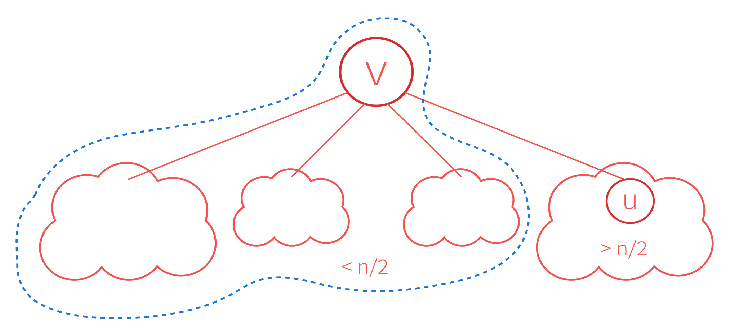We never revisit a node because when we decided to move away from it to a node with subtree size greater than n/2, we sort of declared that it now belongs to the component with nodes less than n/2, and we shall never find our centroid there.
In any case we are moving towards the centroid. Also, there are finitely many vertices in the tree. The process must stop, and it will, at the desired vertex.

Algorithm :

1. Select arbitrary node v
2. Start a DFS from v, and setup subtree sizes
3. Re-position to node v (or start at any arbitrary v that belongs to the tree)
4. Check mathematical condition of centroid for v
1. If condition passed, return current node as centroid
2. Else move to adjacent node with ‘greatest’ subtree size, and back to step 4

Theorem: Given a tree with n nodes, the centroid always exists.
Proof: Clear from our approach to the problem that we can always find a centroid using above steps.

Time Complexity

1. Select arbitrary node v: O(1)
2. DFS: O(n)
3. Reposition to v: O(1)
4. Find centroid: O(n)

Centroid Decomposition :
Finding the centroid for a tree is a part of what we are trying to achieve here. We need to think how can we organize the tree into a structure that decreases the complexity for answering certain ‘type’ of queries.

Algorithm

1. Make the centroid as the root of a new tree (which we will call as the ‘centroid tree’)
2. Recursively decompose the trees in the resulting forest
3. Make the centroids of these trees as children of the centroid which last split them.

The centroid tree has depth O(log n), and can be constructed in O(n lg n), as we can find the centroid in O(n).

Illustrative Example
Let us consider a tree with 16 nodes. The figure has subtree sizes already set up using a DFS from node 1.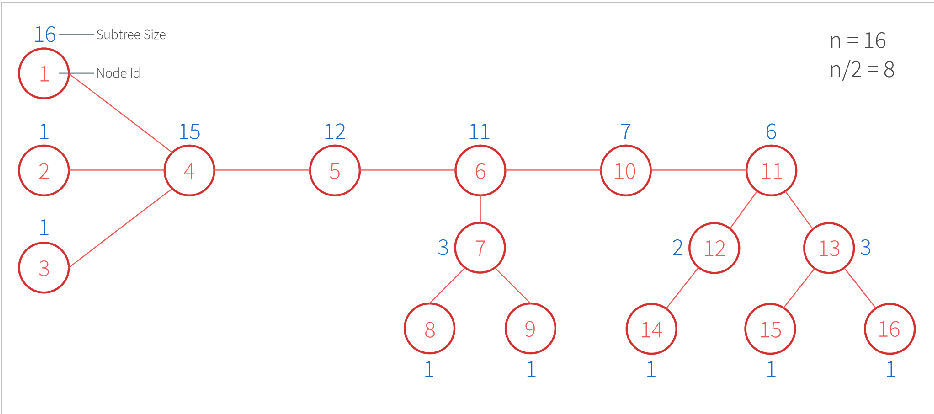We start at node 1 and see if condition for centroid holds. Remember S(v) is subtree size for v.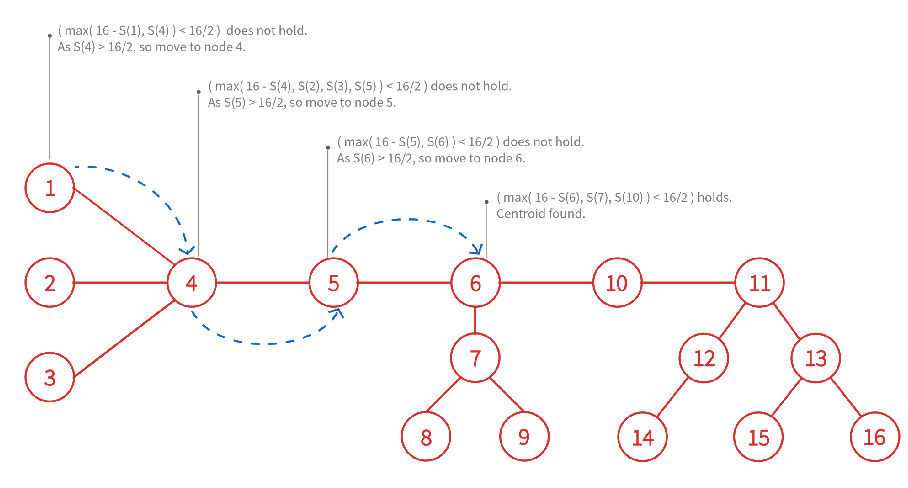We make node 6 as the root of our centroid, and recurse on the 3 trees of the forest centroid split the original tree into.
NOTE: In the figure, subtrees generated by a centroid have been surrounded by a dotted line of the same color as the color of centroid.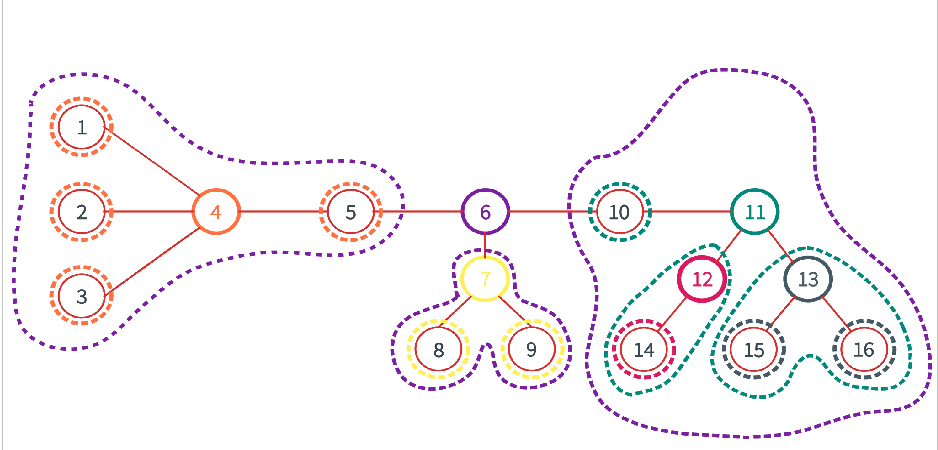We make the subsequently found centroids as the children to centroid that split them last, and obtain our centroid tree.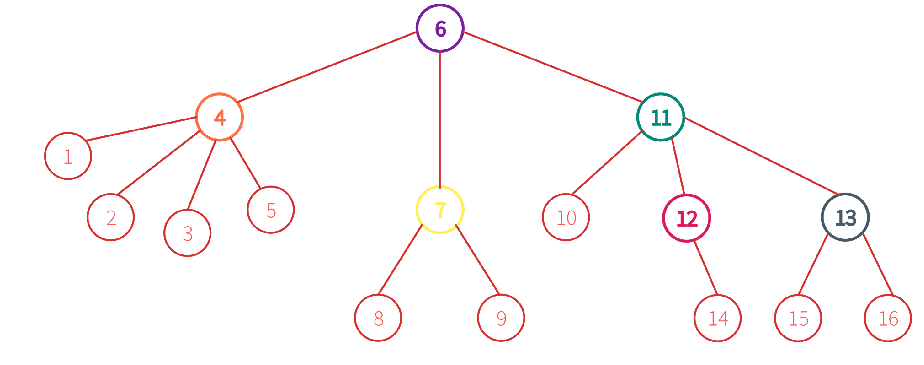NOTE: The trees containing only a single element have the same element as their centroid. We haven’t used color differentiation for such trees, and the leaf nodes represent them.

## C++

 `// C++ program for centroid decomposition of Tree``#include ``using` `namespace` `std;` `#define MAXN 1025` `vector<``int``> tree[MAXN];``vector<``int``> centroidTree[MAXN];``bool` `centroidMarked[MAXN];` `/* method to add edge between to nodes of the undirected tree */``void` `addEdge(``int` `u, ``int` `v)``{``    ``tree[u].push_back(v);``    ``tree[v].push_back(u);``}` `/* method to setup subtree sizes and nodes in current tree */``void` `DFS(``int` `src, ``bool` `visited[], ``int` `subtree_size[], ``int``* n)``{``    ``/* mark node visited */``    ``visited[src] = ``true``;` `    ``/* increase count of nodes visited */``    ``*n += 1;` `    ``/* initialize subtree size for current node*/``    ``subtree_size[src] = 1;` `    ``vector<``int``>::iterator it;` `    ``/* recur on non-visited and non-centroid neighbours */``    ``for` `(it = tree[src].begin(); it!=tree[src].end(); it++)``        ``if` `(!visited[*it] && !centroidMarked[*it])``        ``{``            ``DFS(*it, visited, subtree_size, n);``            ``subtree_size[src]+=subtree_size[*it];``        ``}``}` `int` `getCentroid(``int` `src, ``bool` `visited[], ``int` `subtree_size[], ``int` `n)``{``    ``/* assume the current node to be centroid */``    ``bool` `is_centroid = ``true``;` `    ``/* mark it as visited */``    ``visited[src] = ``true``;` `    ``/* track heaviest child of node, to use in case node is``       ``not centroid */``    ``int` `heaviest_child = 0;` `    ``vector<``int``>::iterator it;` `    ``/* iterate over all adjacent nodes which are children``       ``(not visited) and not marked as centroid to some``       ``subtree */``    ``for` `(it = tree[src].begin(); it!=tree[src].end(); it++)``        ``if` `(!visited[*it] && !centroidMarked[*it])``        ``{``            ``/* If any adjacent node has more than n/2 nodes,``             ``* current node cannot be centroid */``            ``if` `(subtree_size[*it]>n/2)``                ``is_centroid=``false``;` `            ``/* update heaviest child */``            ``if` `(heaviest_child==0 ||``                ``subtree_size[*it]>subtree_size[heaviest_child])``                ``heaviest_child = *it;``        ``}` `    ``/* if current node is a centroid */``    ``if` `(is_centroid && n-subtree_size[src]<=n/2)``        ``return` `src;` `    ``/* else recur on heaviest child */``    ``return` `getCentroid(heaviest_child, visited, subtree_size, n);``}` `/* function to get the centroid of tree rooted at src.`` ``* tree may be the original one or may belong to the forest */``int` `getCentroid(``int` `src)``{``    ``bool` `visited[MAXN];` `    ``int` `subtree_size[MAXN];` `    ``/* initialize auxiliary arrays */``    ``memset``(visited, ``false``, ``sizeof` `visited);``    ``memset``(subtree_size, 0, ``sizeof` `subtree_size);` `    ``/* variable to hold number of nodes in the current tree */``    ``int` `n = 0;` `    ``/* DFS to set up subtree sizes and nodes in current tree */``    ``DFS(src, visited, subtree_size, &n);` `    ``for` `(``int` `i=1; i::iterator it;` `    ``/* for every node adjacent to the found centroid``     ``* and not already marked as centroid */``    ``for` `(it=tree[cend_tree].begin(); it!=tree[cend_tree].end(); it++)``    ``{``        ``if` `(!centroidMarked[*it])``        ``{``            ``/* decompose subtree rooted at adjacent node */``            ``int` `cend_subtree = decomposeTree(*it);` `            ``/* add edge between tree centroid and centroid of subtree */``            ``centroidTree[cend_tree].push_back(cend_subtree);``            ``centroidTree[cend_subtree].push_back(cend_tree);``        ``}``    ``}` `    ``/* return centroid of tree */``    ``return` `cend_tree;``}` `// driver function``int` `main()``{``    ``/* number of nodes in the tree */``    ``int` `n = 16;` `    ``/* arguments in order: node u, node v``     ``* sequencing starts from 1 */``    ``addEdge(1, 4);``    ``addEdge(2, 4);``    ``addEdge(3, 4);``    ``addEdge(4, 5);``    ``addEdge(5, 6);``    ``addEdge(6, 7);``    ``addEdge(7, 8);``    ``addEdge(7, 9);``    ``addEdge(6, 10);``    ``addEdge(10, 11);``    ``addEdge(11, 12);``    ``addEdge(11, 13);``    ``addEdge(12, 14);``    ``addEdge(13, 15);``    ``addEdge(13, 16);` `    ``/* generates centroid tree */``    ``decomposeTree(1);` `    ``return` `0;``}`

Output :

`6 4 1 2 3 5 7 8 9 11 10 12 14 13 15 16`

Application:

Consider below example problem

```Given a weighted tree with N nodes,  find the minimum number
of edges in a path of length K, or return -1 if such a path
does not exist.
1 <= N <= 200000
1 <= length(i;j) <= 1000000 (integer weights)
1 <= K <= 1000000 ```

Brute force solution: For every node, perform DFS to find distance and number of edges to every other node
Time complexity: O(N2) Obviously inefficient because N = 200000

We can solve above problem in O(N Log N) time using Centroid Decomposition.

1. Perform centroid decomposition to get a “tree of subtrees”
2. Start at the root of the decomposition, solve the problem for each subtree as follows
1. Solve the problem for each “child tree” of the current subtree.
2. Perform DFS from the centroid on the current subtree to compute the minimum edge count for paths that include the centroid
1. Two cases: centroid at the end or in the middle of the path

Time complexity of centroid decomposition based solution is O(n log n)

This article is contributed by Yash Varyani. If you like GeeksforGeeks and would like to contribute, you can also write an article and mail your article to review-team@geeksforgeeks.org. See your article appearing on the GeeksforGeeks main page and help other Geeks.